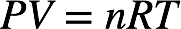# Problem: A weather balloon is inflated to a volume of 28.5 L at a pressure of 748 mmHg and a temperature of 28.0 °C. The balloon rises in the atmosphere to an altitude of approximately 25,000 feet, where the pressure is 385 mmHg and the temperature is -15.0 °C. Assuming the balloon can freely expand, calculate the volume of the balloon at this altitude.

###### FREE Expert Solution
79% (172 ratings)
###### FREE Expert Solution

Initially, a weather balloon has a volume of 28.5 L at 28.0 ˚C and 748 mmHg. We’re being asked to determine the volume of the weather balloon if the pressure changes to 385 mmHg and the temperature changes to –15.0 ˚C.

Recall that the ideal gas law is:The pressure, volume, and temperature of a gas are related to the number of moles of gas and the universal gas constant:The value nR is constant. For a given moles of gas, the initial and final pressure, volume, and temperature of the gas are related by the combined gas law:79% (172 ratings)###### Problem Details

A weather balloon is inflated to a volume of 28.5 L at a pressure of 748 mmHg and a temperature of 28.0 °C. The balloon rises in the atmosphere to an altitude of approximately 25,000 feet, where the pressure is 385 mmHg and the temperature is -15.0 °C. Assuming the balloon can freely expand, calculate the volume of the balloon at this altitude.

What scientific concept do you need to know in order to solve this problem?

Our tutors have indicated that to solve this problem you will need to apply the Chemistry Gas Laws concept. If you need more Chemistry Gas Laws practice, you can also practice Chemistry Gas Laws practice problems.

What is the difficulty of this problem?

Our tutors rated the difficulty ofA weather balloon is inflated to a volume of 28.5 L at a pre...as high difficulty.

What professor is this problem relevant for?

Based on our data, we think this problem is relevant for Professor Parnis' class at Trent University.

What textbook is this problem found in?

Our data indicates that this problem or a close variation was asked in Chemistry: A Molecular Approach - Tro 2nd Edition. You can also practice Chemistry: A Molecular Approach - Tro 2nd Edition practice problems.